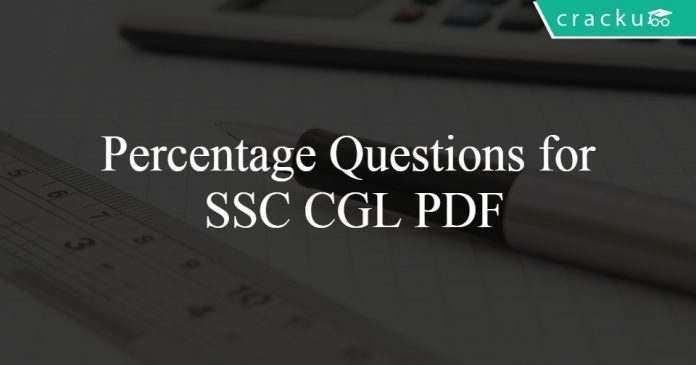# Percentage Questions for SSC CGL PDF

1
12209Percentage Questions for SSC CGL PDF

# Percentage Questions for SSC CGL PDF:

Question 1: If 30% of A is added to 40% of B, the answer is 80% of B. What percentage of A is B?

a) 30%
b) 40%
c) 70%
d) 75%

Question 2: A man spends 59% of his income and saves the rest. What percentage of his expenditure does he save?

a) 72.57 percent
b) 83.19 percent
c) 89.09 percent
d) 69.49 percent

Question 3: A grain dealer cheats to the extent of 10% while buying as well as selling by using false weights. His total profit percentage is :

a) 20%
b) 22.22%
c) 23.23%
d) 25%

Question 4: The costs of daily ticket of local train is Rs 130 and Monthly Pass costs Rs 3091.40. If I buy the Monthly Pass and travel for 29 days in a month than I save (in percentage)?

a) 16 percent
b) 18 percent
c) 15 percent
d) 14 percent

Question 5: The ratio of the number of boys and girls in a school is 2 : 3. If 25% of the boys and 30% of the girls are scholarship holders, the percentage of the school students who are not scholarship holders is

a) 72
b) 36
c) 54
d) 60

Question 6: The percentage increase in the area of a rectangle, if each of its sides is increased by 20% is equal to

a) 32%
b) 34%
c) 42%
d) 44%

Question 7: A man spends 75% of his income. His income increases by 20% and his expenditure also increases by 10%. The percentage of increase in his savings is

a) 40%
b) 30%
c) 50%
d) 25%

Question 8: The marked price of a shirt is Rs 1280. If the shirt is being sold for Rs 900, then what is the discount percentage?

a) 31.31
b) 25.57
c) 29.68
d) 34.36

Question 9: The weights of two iron balls are 3.5 kg and 7.5 kg. What is the percentage weight of the 1st ball with respect to 2nd ball.

a) $46\frac{2}{3}4632​%$
b) 35%
c) $46\frac{1}{3}4631​%$
d) 45%

Question 10: A student multiplied a number by 5/6 instead of 6/5. What is the percentage error in the calculation?

a) 44 percent
b) 30.56 percent
c) 15.28 percent
d) 22 percent

Answers & Solutions for Percentage Questions for SSC CGL PDF:

1) Answer (D)
it is given that
0.3 A + 0.4 B = 0.8 B
0.3 A = 0.4 B
A = 4/3 B
B = 3/4 A
hence B is 75 % of A

2) Answer (D)
Let total income of man = Rs. 100x
Amount spent = $\frac{59}{100} \times 100x = Rs. 59x$
=> Money saved = $100x – 59x = 41x$
∴ Percentage of his expenditure that he saves = $\frac{41x}{59x} \times 100$
= 69.49 %

3) Answer (B)
let the retailer buys 110 gram in Rs 100 because of 10 % cheating
so cost price = 1 gram = Rs 100/110 = Rs 0.909
and retailer sells 90 gram in Rs 100
and hence selling price = Rs 100 / 90 = Rs 1.111
Profit % = $\frac{1.111- 0.909}{0.909} = 22.22%$

4)  Answer (B)
Train ticket cost for 1 day = Rs. 130
=> Cost for 29 days = $29 \times× 130 = Rs. 3770$
Cost of monthly pass = Rs. 3091.40
=> Money saved = 3770 – 3091.40 = Rs 678.6
∴ Required % = $\frac{678.6}{3770} \times 100 = 18%$
=> Ans – (B)

5) Answer (A)
Let the number of boys be 2y and number of girls be 3y
25 % of boys = 0.25 x 2y = 0.5y
30% of girls = 0.3 x 3y = 0.9y
total scholarship holder = 0.5y + 0.9y = 1.4y
% of no scholarship holder = $\frac{3.6y}{5y} \times 100 = 72%$

6) Answer (D)
let the length of rectangle be L units and breadth be B units.
So area of rectangle = L x B
As both length and breadth has been increased by 20%
So new length = 1.2L
New breadth = 1.2B
New area = 1.2L x 1.2B = 1.44LB
Percentage increase in area = $\frac{1.44LB – LB}{LB} ​\times 100 = 44%$

7) Answer (C)
let the income of person be Rs y
So his expenditure be 75% of income which is = 0.75y
Saving = y- 0.75y = 0.25y
Now after increment of 20 % in income the new income becomes = 1.2y
Given that bew expenditure is 10% more than previous one.So , new expenditure is = 1.1 × 0.75y = 0.825y
New savings = 1.2y – 0.825y = 0.375y
Percentage increase in savings = $\frac {0.375y-0.25y}{0.25y} \times 100 = 50% 8) Answer (C) Marked price = Rs. 1280 Selling price = Rs. 900 => Discount % =$\frac{1280-900}{1280}\times 100$=$\frac{380}{12.8}=29.68%$9) Answer (A) Weight of 1st ball = 3.5 kg and weight of second ball = 7.5 kg => Percentage weight of the 1st ball with respect to 2nd ball =$\frac{3.5}{7.5} \times 100$=$\frac{35}{3} \times 4$=$\frac{140}{3}$=$46\frac{2}{3}%$10) Answer (B) Let the number be 30 When the student multiplied it by 5/6, => original result =$\frac{5}{6} \times 30 = 25$When the student multiply it by 6/5, => New result =$\frac{6}{5} \times 30 = 36$=> Percentage error in calculation =$\frac{(36 – 25)}{36} \times 100$=$\frac{1100}{36} = 30.56%\$
=> Ans – (B)